# Getting Started with Plotly-Python

The Plotly Python library is an interactive open-source library. This can be a very helpful tool for data visualization and understanding the data simply and easily. plotly graph objects are a high-level interface to plotly which are easy to use. It can plot various types of graphs and charts like scatter plots, line charts, bar charts, box plots, histograms, pie charts, etc.

So you all must be wondering why plotly over other visualization tools or libraries? Here’s the answer –

• Plotly has hover tool capabilities that allow us to detect any outliers or anomalies in a large number of data points.
• It is visually attractive that can be accepted by a wide range of audiences.
• It allows us for the endless customization of our graphs that makes our plot more meaningful and understandable for others.

Ok, enough theory let’s start.

Installation:

To install this module type the below command in the terminal.

`pip install plotly`

### Getting Started

Let’s create various plots using this module

• Scatter Plot:  Scatter plot represent values for two different numeric variables. They are mainly used for the representation of the relationship between two variables.

## Python3

 `# import all required libraries` `import` `numpy as np` `import` `plotly` `import` `plotly.graph_objects as go` `import` `plotly.offline as pyo` `from` `plotly.offline ``import` `init_notebook_mode`   `init_notebook_mode(connected``=``True``)`   `# generating 150 random integers` `# from 1 to 50` `x ``=` `np.random.randint(low``=``1``, high``=``50``, size``=``150``)``*``0.1`   `# generating 150 random integers` `# from 1 to 50` `y ``=` `np.random.randint(low``=``1``, high``=``50``, size``=``150``)``*``0.1`   `# plotting scatter plot` `fig ``=` `go.Figure(data``=``go.Scatter(x``=``x, y``=``y, mode``=``'markers'``))`   `fig.show()`

Output: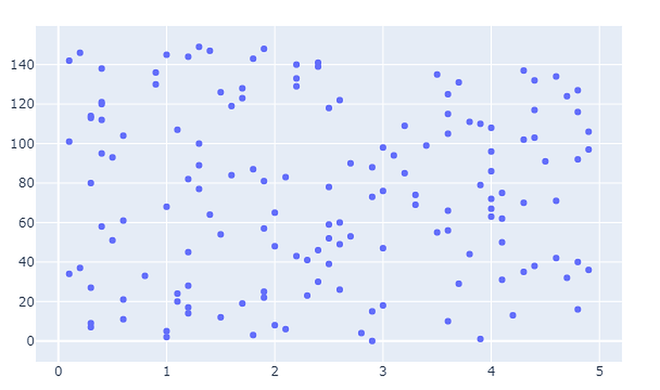• Bar charts: Bar charts are used when we want to compare different groups of data and make inferences of which groups are highest and which groups are common and compare how one group is performing compared to others.

## Python3

 `# import all required libraries` `import` `numpy as np` `import` `plotly` `import` `plotly.graph_objects as go` `import` `plotly.offline as pyo` `from` `plotly.offline ``import` `init_notebook_mode`   `init_notebook_mode(connected ``=` `True``) `   `# countries on x-axis` `countries``=``[``'India'``, ``'canada'``,` `           ``'Australia'``,``'Brazil'``,` `           ``'Mexico'``,``'Russia'``,` `           ``'Germany'``,``'Switzerland'``,` `           ``'Texas'``] `   `# plotting corresponding y for each ` `# country in x ` `fig ``=` `go.Figure([go.Bar(x``=``countries,` `                        ``y``=``[``80``,``70``,``60``,``50``,` `                           ``40``,``50``,``60``,``70``,``80``])]) `   `fig.show()`

Output: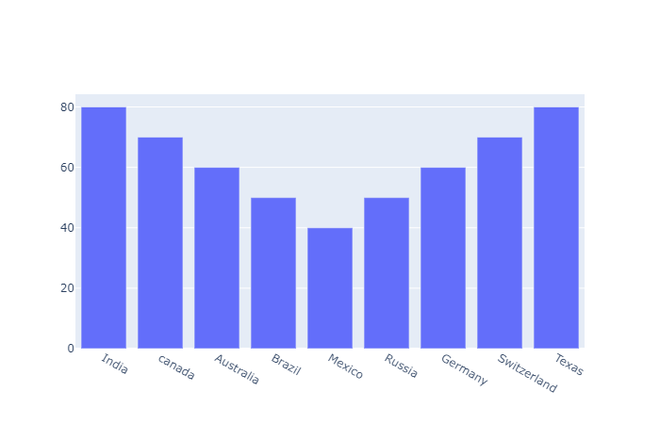• Pie chart: A pie chart represents the distribution of different variables among total. In the pie chart each slice shows its contribution to the total amount.

## Python3

 `# import all required libraries` `import` `numpy as np` `import` `plotly` `import` `plotly.graph_objects as go` `import` `plotly.offline as pyo` `from` `plotly.offline ``import` `init_notebook_mode`   `init_notebook_mode(connected ``=` `True``) `   `# different individual parts in ` `# total chart` `countries``=``[``'India'``, ``'canada'``, ` `           ``'Australia'``,``'Brazil'``,` `           ``'Mexico'``,``'Russia'``,` `           ``'Germany'``,``'Switzerland'``,` `           ``'Texas'``] `   `# values corresponding to each ` `# individual country present in` `# countries` `values ``=` `[``4500``, ``2500``, ``1053``, ``500``,` `          ``3200``, ``1500``, ``1253``, ``600``, ``3500``] `   `# plotting pie chart` `fig ``=` `go.Figure(data``=``[go.Pie(labels``=``countries,` `                      ``values``=``values)])`   `fig.show()`

Output: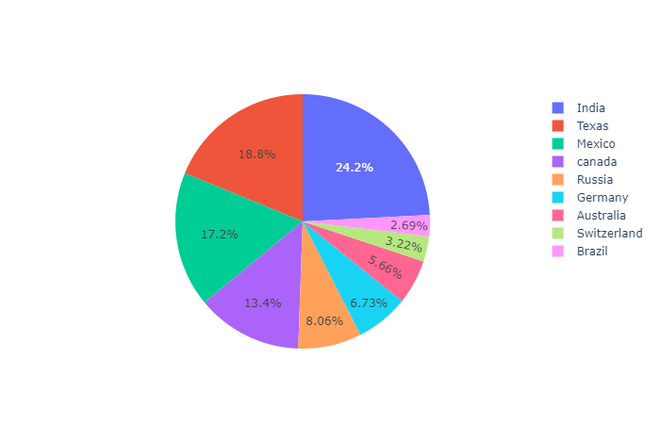• Histogram: A histogram plots the continuous distribution of variable as series of bars and each bar indicates the frequency of the occurring value in a variable. In order to use a histogram, we simply require a variable that takes continuous numeric values

## Python3

 `# import all required libraries` `import` `numpy as np` `import` `plotly` `import` `plotly.graph_objects as go` `import` `plotly.offline as pyo` `from` `plotly.offline ``import` `init_notebook_mode`   `init_notebook_mode(connected ``=` `True``) `   `# save the state of random` `np.random.seed(``42``)  `   `# generating 250 random numbers` `x ``=` `np.random.randn(``250``) `   `# plotting histogram for x` `fig ``=` `go.Figure(data``=``[go.Histogram(x``=``x)]) `   `fig.show()`

Output: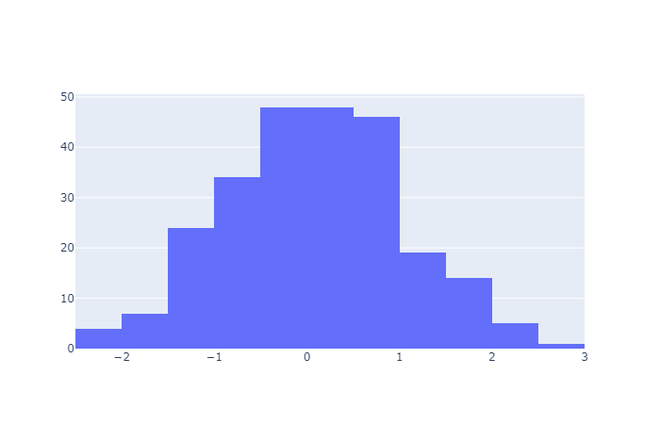• Box plot: A box plot is the representation of a statistical summary. Minimum, First Quartile, Median, Third Quartile, Maximum.

## Python3

 `# import all required libraries` `import` `numpy as np` `import` `plotly` `import` `plotly.graph_objects as go` `import` `plotly.offline as pyo` `from` `plotly.offline ``import` `init_notebook_mode`   `init_notebook_mode(connected ``=` `True``) `   `np.random.seed(``42``)`   `# generating 50 random numbers` `y ``=` `np.random.randn(``50``) `   `# generating 50 random numbers` `y1 ``=` `np.random.randn(``50``) ` `fig ``=` `go.Figure()  `   `# updating the figure with y` `fig.add_trace(go.Box(y``=``y)) `   `# updating the figure with y1` `fig.add_trace(go.Box(y``=``y1)) `   `fig.show()`

Output: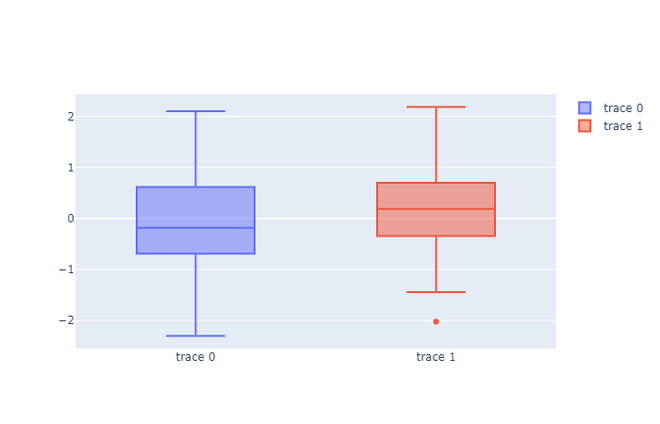Whether you're preparing for your first job interview or aiming to upskill in this ever-evolving tech landscape, GeeksforGeeks Courses are your key to success. We provide top-quality content at affordable prices, all geared towards accelerating your growth in a time-bound manner. Join the millions we've already empowered, and we're here to do the same for you. Don't miss out - check it out now!

Previous
Next